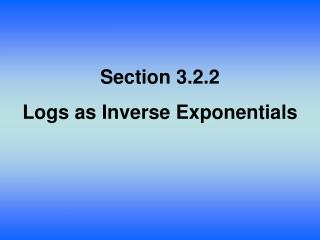DownloadDownload PresentationSection 3.2.2 Logs as Inverse Exponentials

# Section 3.2.2 Logs as Inverse Exponentials

Télécharger la présentation## Section 3.2.2 Logs as Inverse Exponentials

- - - - - - - - - - - - - - - - - - - - - - - - - - - E N D - - - - - - - - - - - - - - - - - - - - - - - - - - -
##### Presentation Transcript

1. Section 3.2.2 Logs as Inverse Exponentials

2. Lesson Objective: Students will: • Redevelop the log function by reversing a table for an exponential function. • Practice converting between exponential and log equations. • Formally define the log function.

3. Vocabulary: Logarithm

4. Closure What is a logarithm? When the base is not shown in a logarithm, what is it assumed to be?

5. Closure Write a logarithmic equation for Write an exponential equation for What is the inverse to an exponential function called?

6. Assignment Pg 152#3-73 to 3-81